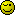# Fill PHP array from mySQL table

I am trying to use a mysql query to fill a PHP array but it doesn’t seem to work correctly. Here is the code I am using now:

``````\$a = array();
\$rt = mysql_query("SELECT * FROM users WHERE upline='{\$ir['username']}' AND uplinetype=2");
while(\$r=mysql_fetch_array(\$rt))
{
for(\$count=0; \$count < \$ir['referrals']; \$count++)
{
}
}

foreach(\$a as \$value) {
echo "\$value\
";
}
``````

the \$ir array is defined elsewhere and I know this isn’t the problem. Am I filling the array wrong or is something wrong with the code? Any help is appreciated. Thanks.

Your request is a little strange, but this should work…

``````<?php
\$aArray = array();
\$sSQL = sprintf("SELECT username FROM users WHERE upline='&#37;s' AND uplinetype=2",
);
\$rResult = mysql_query(\$sSQL);
while (\$aRow = mysql_fetch_assoc(\$rResult))
{
}
?>
``````

Hmm this seems to print only one value?

I am using this:

``````\$aArray = array();
\$sSQL = sprintf("SELECT username FROM users WHERE upline='&#37;s' AND uplinetype=2",
);
\$rResult = mysql_query(\$sSQL);
while (\$aRow = mysql_fetch_assoc(\$rResult))
{
}

foreach(\$aArray as \$value) {
echo "\$value\
";
}
``````

It only prints one result when it should print 3

That’s all your original script would have done, what are looking to achieve?

I want to print everything that the mysql query returns and store those values in an array. There should be multiple values where upline="\$ir[‘username’] and uplinetype=‘2’.

OK, give this a whirl…

``````
<?php
\$aArray = array();
\$sSQL = sprintf("SELECT * FROM users WHERE upline='&#37;s' AND uplinetype=2",
);
\$rResult = mysql_query(\$sSQL);
while (\$aRow = mysql_fetch_assoc(\$rResult))
{
\$aArray[] = \$aRow;
}
print_r(\$aArray);
?>

``````

Thank you so much!!! I really appreciate it!

No problem, glad we finally got to the bottom of it for you.Okay, one more question. I am not sure if you’re able to do this but: Is there a way I can have a WHERE statement in mysql query where I can compare a table field to the array I just created?

Do you have an example?

Something like:

``````mysql_query("UPDATE users SET upline='{\$mainuser['username']}', uplinetype='2',
totalclicks>".round(\$set['refs_minclicks'])." AND lastaction>{\$tsp} AND
``````

Where \$aArray is what I(well, you :D) just created.

So you only want to select 1 username from the array that was created? If so, how would you determine which one?

Or do you want to exclude all users that were found in the previous query, which are now contained in the array?

I want to select a single user that is NOT from the array that I just created.

This query should do what you ask without using the array we created.

``````
<?php
\$sSQL = sprintf("UPDATE users SET upline = '&#37;s', uplinetype = 2, uplineremains = '%s' WHERE username != '%s' AND username != '%s' AND upline = '' AND totalclicks > %s AND lastaction > %s AND username NOT IN (SELECT username FROM users WHERE upline='%s' AND uplinetype = 2) LIMIT 1;",
\$data['uplineremains'],
Good luck, and I’m sure the guys over there will sort you out.